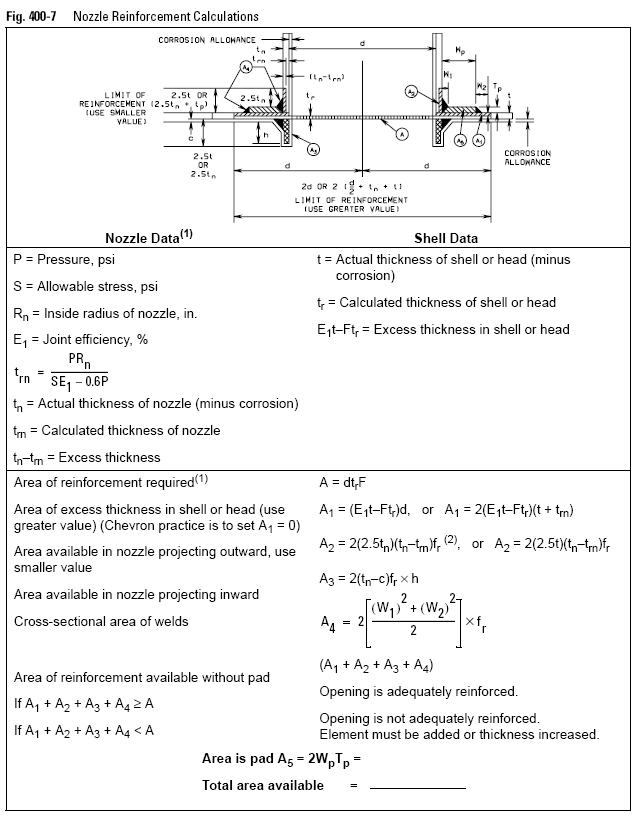﻿ Calculations for Reinforcement of Openings – Oil & Gas Industry Technology Updates

## Calculations for Reinforcement of Openings

To determine whether an opening is adequately reinforced, it is first necessary to
determine whether the areas of reinforcement available will be sufficient without the
use of a pad. The total cross-sectional area of reinforcement required (in square
inches) is indicated by the letter A, which is equal to the diameter (plus corrosion
allowance) times the required thickness. The area of reinforcement available

• The area of excess thickness in the shell or head, A1 (Usual practice is to set A1 = 0 and allocate it to corrosion allowance.)
• The area of excess thickness in the nozzle wall, A2
• The area available in the nozzle projecting inward, A3
• The cross-sectional area of welds, A4

If A1 + A2 + A3 + A4 ³ A, the opening is adequately reinforced. If A1 + A2 + A3 + A4 < A, a pad is needed.

If the reinforcement is found to be inadequate, then the area of pad needed (A5) may be calculated as follows:

A5 = A – (A1 + A2 + A3 + A4)
(Eq. 400-25)

See Figure 400-7 for nozzle and shell data, equations, and nomenclature for calculating the area of pad needed for reinforcement.If a pad is used, the factor (2.5t) in the equation for A2 in Figure 400-7 is measured from the top surface of the pad and, therefore, becomes (2.5tn + Tp). The area A2 must be recalculated on this basis and the smaller value again used. Then:

If A1 + A2 + A3 + A4 + A5 >= A, the opening is adequately reinforced.

The other symbols in Figure 400-7 for the area equations (all values except E1, and F are in inches) are as follows:
d = Diameter in the plane under consideration of the finished opening in its corroded condition
t = Nominal thickness of shell or head, less corrosion allowance

tr = Required thickness of shell or head as defined in Code Paragraph UG-37
trn = Required thickness of a seamless nozzle wall
Tp = Thickness of reinforcement pad
Wp = Width of reinforcement pad
tn = Nominal thickness of nozzle wall, less corrosion allowance
W1 = Cross-sectional area of weld
W2 = Cross-sectional area of weld
E1 = 1 when an opening is in the plate or when the opening passes through a circumferential point in a shell or cone (exclusive of head-to-shell joints), or Joint efficiency obtained from Code Table UW-12 when any part of the opening passes through any other welded joint
F = Correction factor which compensates for the variation in pressure stresses on different planes with respect to the axis of a vessel. A value of 1.00 is used for F in all cases except when the opening is integrally reinforced. If integrally reinforced, see Code Figure UG-37.1
fr = Strength reduction factor. Ratio of material stresses: £ 1.0
h = Distance nozzle projects beyond the inner surface of the vessel wall before corrosion allowance is added.

To correct for corrosion, deduct the specified allowance from shell thickness, t, and nozzle thickness, tn, but add twice its value to the diameter of opening, d. Note Total load to be carried by attachment welds may be calculated from the equation, W = S(A-A1), in which S equals the allowable stress specified by Code Section C and Code Paragraph UW-15(b). For large openings in cylindrical shells, see Code Appendices 1-7. For openings in flat heads, see Code Paragraph UG-39. For weld sizes required, see Code Paragraph UW-16 and Code Figure UW-16.1.

On all welded vessels built under column C of Code Table UW-12, 80% of the allowable stress value must be used in design equations and calculations.